# Visualizing Variance and Standard Deviation

So this wasn’t on today’s to-do list, but there seems to be a cash prize associated with this rabbit hole due to this tweet:I generally fall in the camp of people who are skeptical of graduate-level journalism schools, but I would absolutely pay Columbia University \$98,000 if it could teach me how to clearly and concisely translate “one standard deviation from the mean” for regular readers — Max Fisher (@Max_Fisher) August 13, 2018 Note: Tweet has been deleted; the text is from my blogpost when the tweet was still active. Updating Sept 18, 2018

And communicating about standard deviations. That’s like one of my favorite topics! The project is now on my to-do list.

Full disclosure, I’ve already written on this topic here: From N to Standard Deviation: Visualizing Univariate Statistics. The current post was be a matter of focusing on variance and sd, and clean-ups as any messes were encountered (encountered they were!). One more thing: the calculation is for population variance and sd.

So here we go. I’m using the gapminder dataset which is ever-so-handy as it’s available in an R package (thanks Jenny Bryan). For the exercise I’ll just be looking at European countries in 2007, and focusing exclusively on the life expectancy variable.

Let’s look at a plot of the data.Cool. So what are the variance and standard deviation for life expectancy of European Countries in 2007?

Well, the equation for variance is as follows.

$\sigma^2 = \frac{\sum_{i=1}^{n}(x_i - \mu)^2} {n}$

Can you mentally visualize it? Maybe not? Let’s walk through together then.

First we need the mean, $\mu$. We plot it in red on the figure.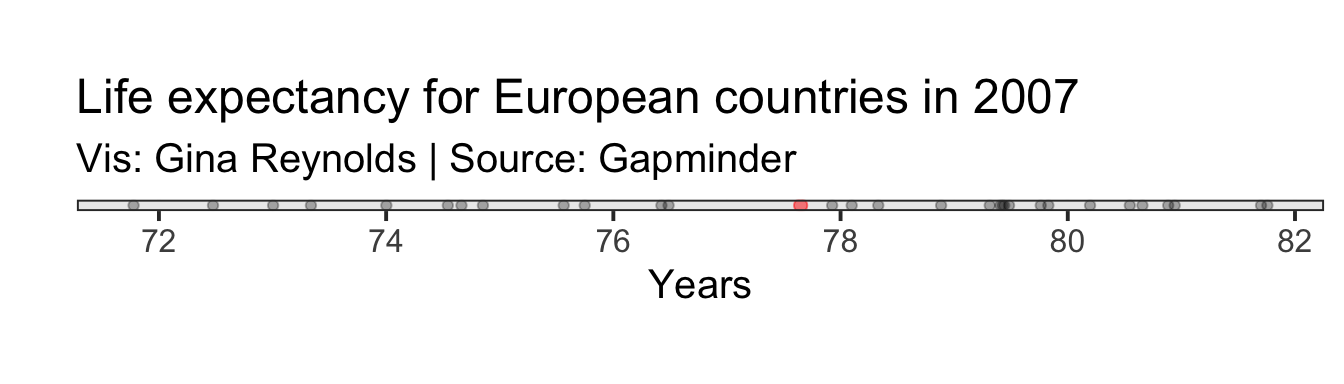Then we do an operation for each of our observations, $(x_i - \mu)^2$. In words, for an observation, say Romania, take the difference for its life expectancy value from the mean. Then square that. So, let’s just do that for one of our observations. Our Romania example will work fine. We plot the point representing Romania in green.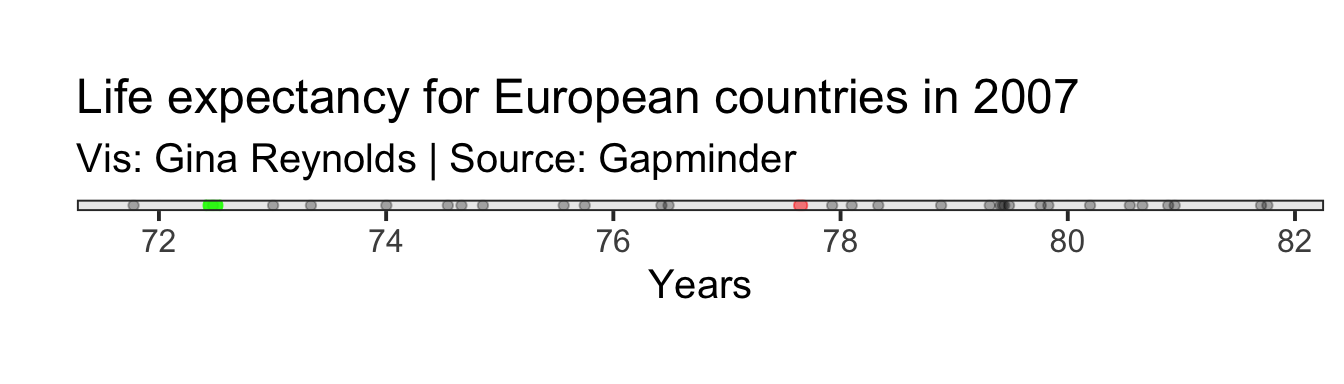Now take the difference between the mean for all observations, $\mu$, and the life expectancy for Romania.

Calculation for Romania: $(x_{Romania} - \mu)$

This difference is shown with the green line: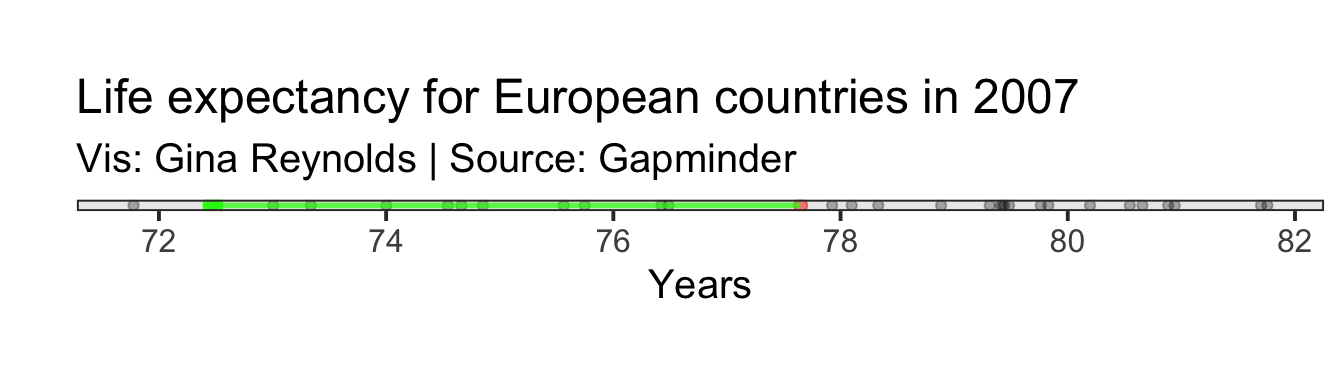Variance: $\sigma^2 = \frac{\sum_{i=1}^{n}(x_i - \mu)^2} {n}$

Then we need to square that difference. This resultant value is equal to the area of a square that has as one of its sides the difference in life expectancy for Romania and the mean life expectancy for all observations. It is a transparent green square in the plot.

Calculation for Romania: $(x_{Romania} - \mu)^2$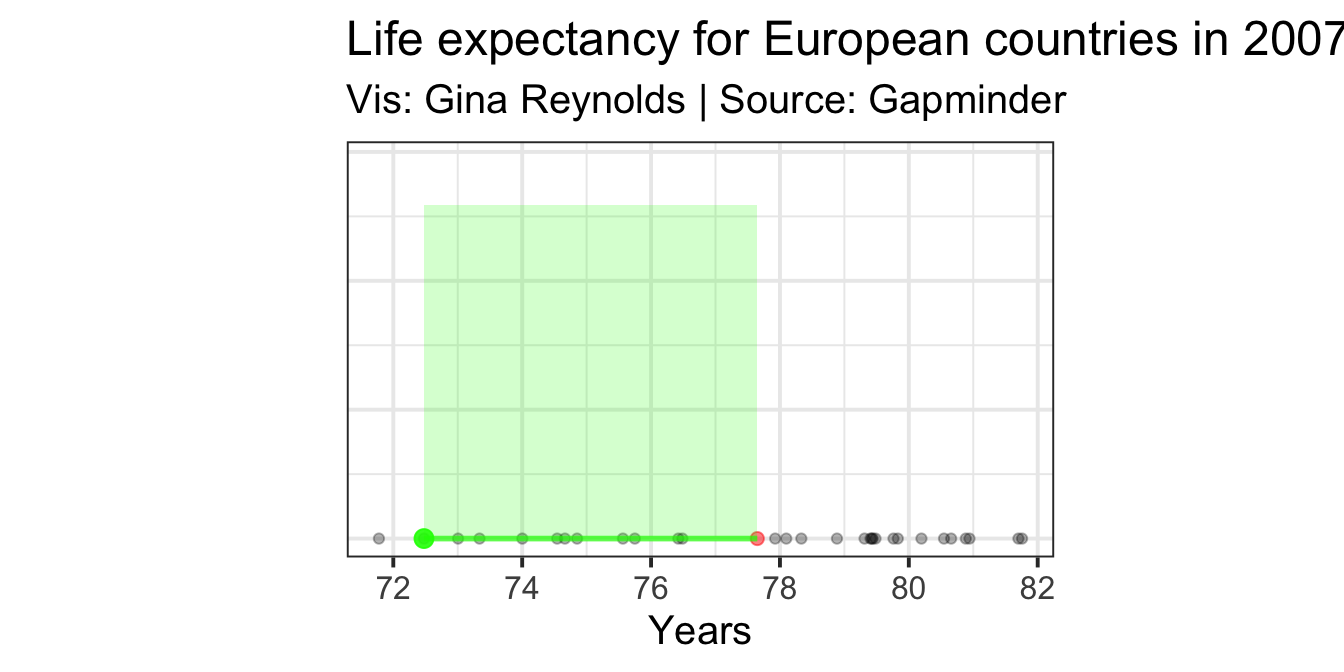Variance: $\sigma^2 = \frac{\sum_{i=1}^{n}(x_i - \mu)^2} {n}$

Now we need to do the same for all of our observations. Not just Romania, but also Germany, Italy, Sweden, France, and so on.

Doing that we get the result (Yup, Romania’s still there in green):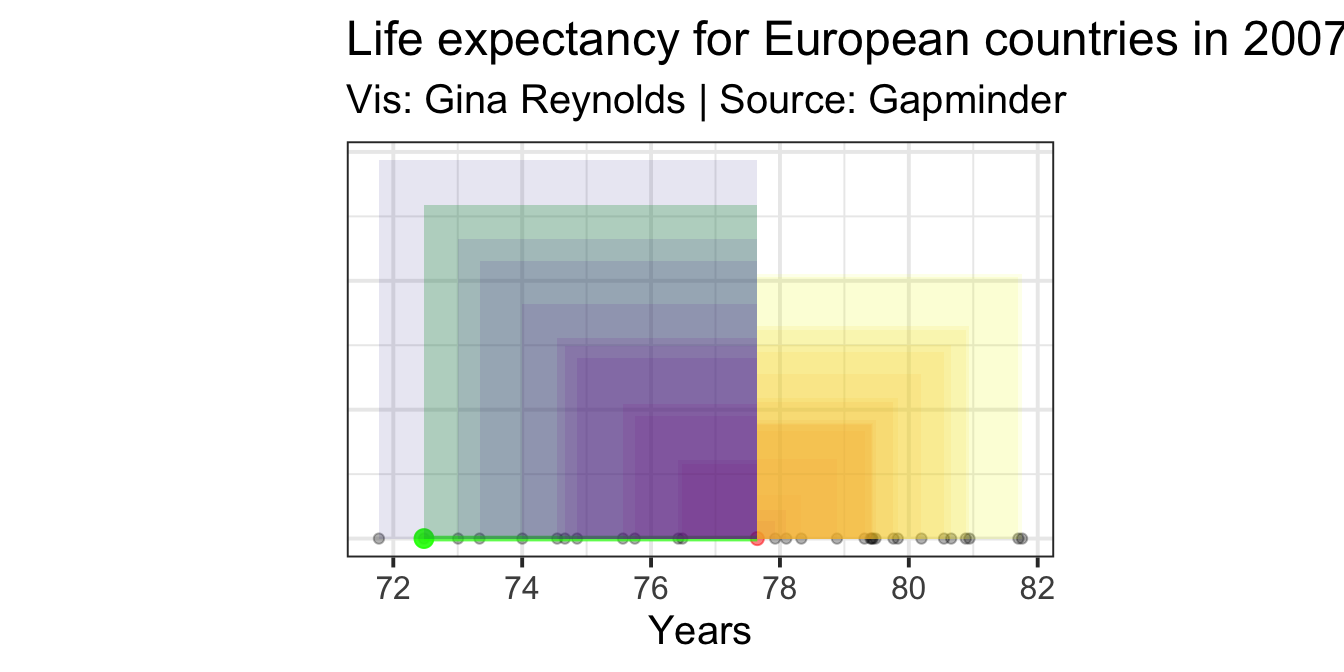The average of all of these areas is the variance. We simply sum up all of the resulting areas that are shown in the plot (note that these areas are overlapping), and divide by the number of these squares:

$\sum_{i=1}^{n}(x_i - \mu)^2/n$

The result is the variance. It is equivalent to the area of the orange square in the plot below. Again, it is simply the average area of all of the squares we created previously. The corner of the orange square, whose area is the variance, happens to be at the mean value, but it need not be so.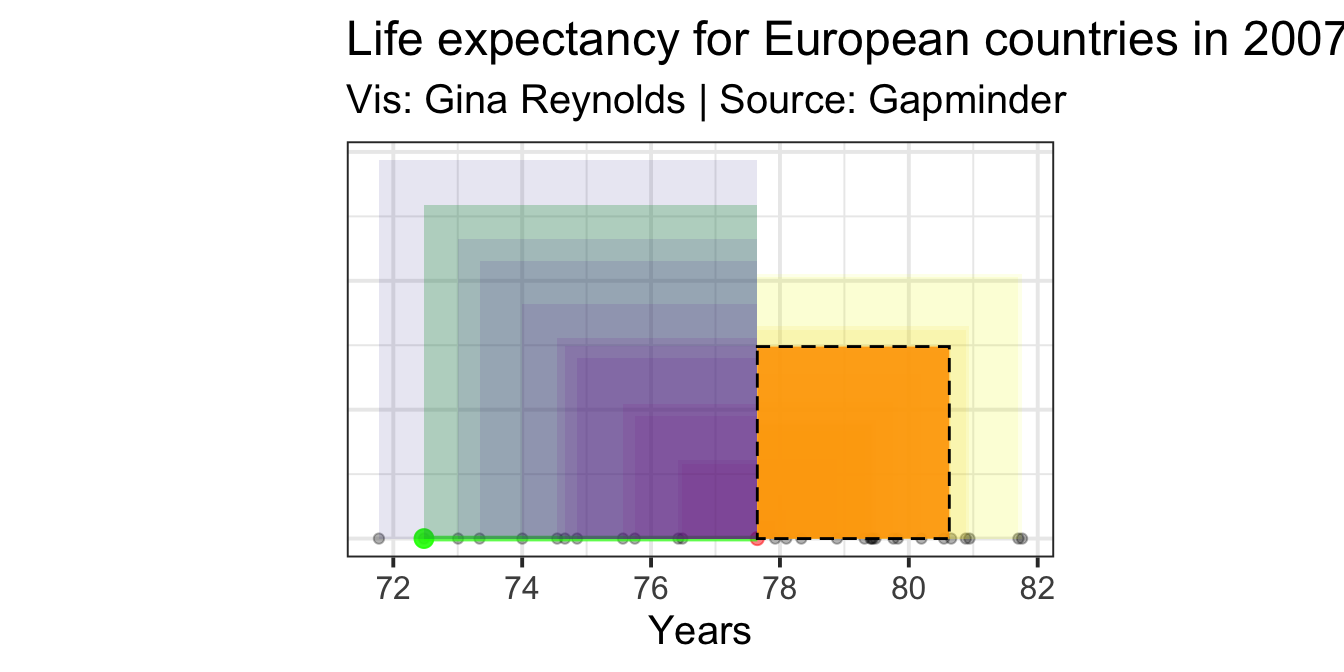# Standard Deviation:

Now, calculating the standard deviation is straightforward. Take the square root of the variance (that orange square).

Standard Deviation: $\sigma = \sqrt\frac{\sum_{i=1}^{n}(x_i - \mu)^2} {n}$

The standard deviation on the plot can be represented as simply the length of the edge of the square whose area is the variance (i.e. the length of the side of the bright orange square). The standard deviation (the resulting length) is highlighted in blue on the plot: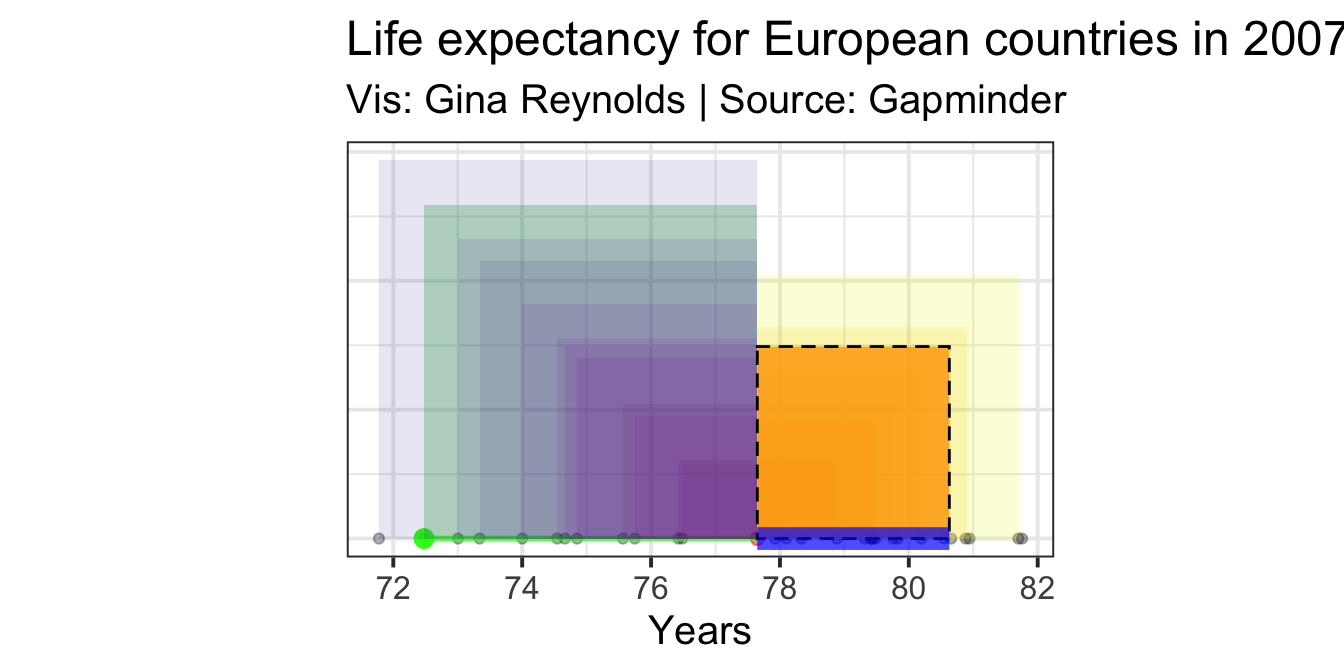That’s it! I hope that this will help you visualize variance and sd in the future.

Discussion question(s):

What do you think of as the first step to finding the variance and standard deviation? Which measure do you think feels more useful as a description of spread of a variable? What are the units of each?

## Quick final note:

We could equivalently represent the squared differences (observation value - mean squared) in a bar chart: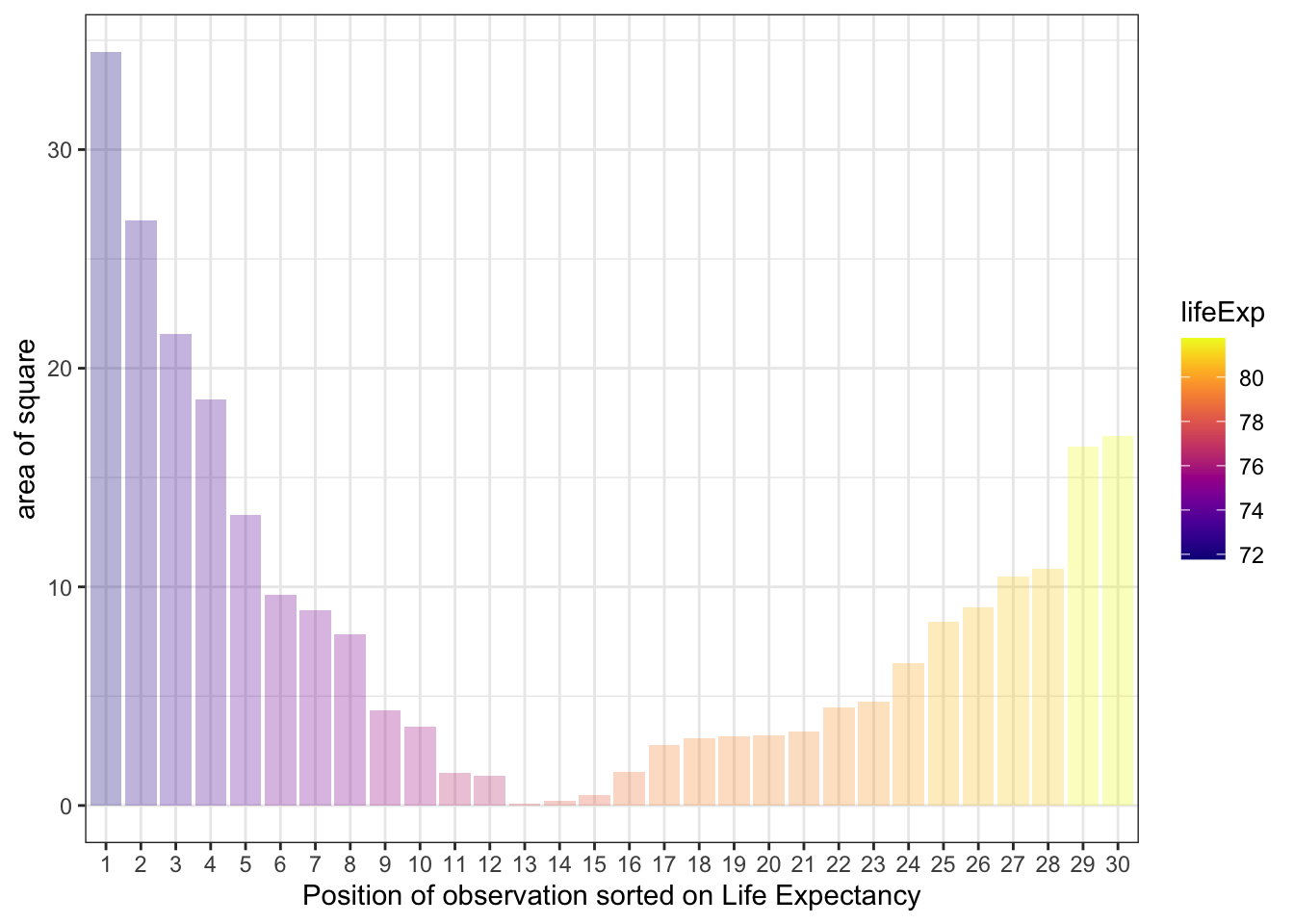And then we divide through by the number of observations (e.g. the total number of squares) which gives us our aim - the variance, represented as $\sigma^2$:

$\sigma^2 = \frac{\sum_{i=1}^{n}(x_i - \mu)^2} {n}$

And taking the average gives us the level of the dotted line and grey bars: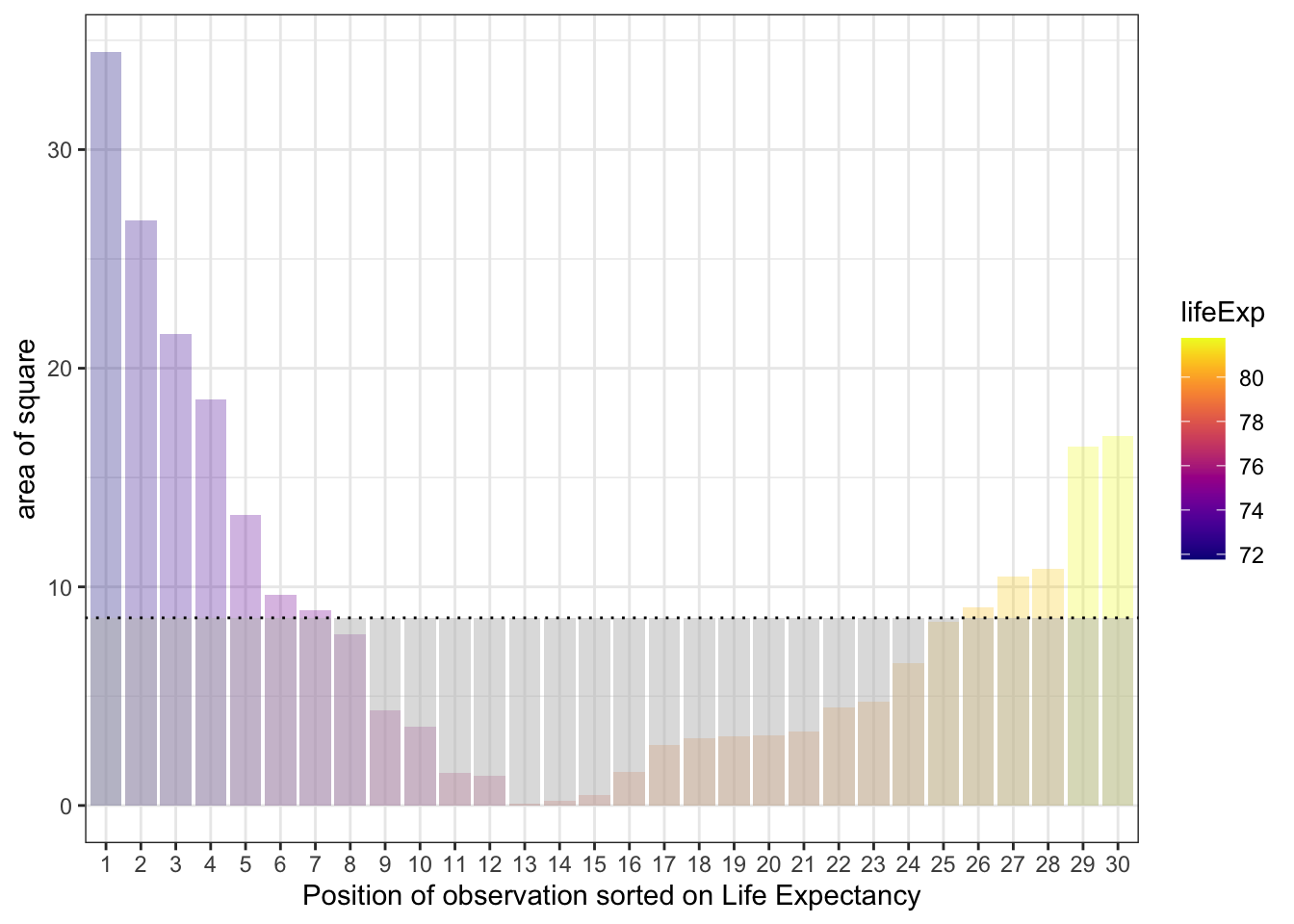So that is the variance: the mean of the areas of the squares that have edges that are the distance between observation values and the population mean value.

The standard deviation is is the length of the edge of the square that has the area which is the variance.

And these two last sentences are the reason I like to keep things step-by-step and visual!

##### Evangeline Reynolds
###### Visiting Teaching Assistant Professor

My research interests include international institutions, causal inference, data visualization, and computational social science and pedagogy.